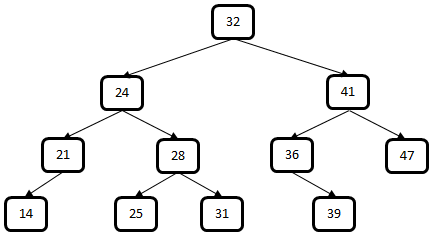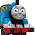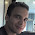## Sunday, March 10, 2013

### No. 46 - Nodes with Sum in a Binary Search Tree

Problem: Given a binary search tree, please check whether there are two nodes in it whose sum equals a given value.
﻿Figure 1: A sample binary search tree

For example, if the given sum is 66, there are two nodes in Figure 1 with value 25 and 41 whose sum is 66. While the given sum is 58, there are not two nodes whose sum is same as the given value.

Analysis: In a previous blog, we discussed how to check whether there are two numbers in a sorted array whose sum equals a given value. In order to solve this problem, we initialize a number num1 as the smallest number in the array, and initialize num2 as the greatest one. If the sum of num1 and num2 is same as the given value, two required numbers have been found. If the sum is greater than the given value, we move num2 to the previous number in the array (with less value). Similarly, we move num1 to the next number in the array (with greater value) if the sum is less than the given value.

Inspired by the solution, we may find two nodes with a given sum in a binary search tree with similar strategy. Firstly we initialize a pointer P1 to the smallest node in the tree, and another pointer P2 to the greatest node. When the sum of two values in the nodes pointed by P1 and P2 is same as the given value, two required nodes have been found. If their sum is greater than the given value, we move P2 to the previous node (containing less value). Moreover, we move P1 to the next node (containing greater value) if their sum is less than the given value.

It is not difficult to get the least and greatest value of a binary search tree. If we move along the links to left children, and the leaf node we arrive at finally contains the least value. For instance, if we move along the links to left children in Figure 1, the nodes on the path contain value 32, 24, 21 and 14, and 14 is the least value in the binary search tree.

Similarly, if we move along links to right children, and the leaf node we arrive at finally contains the greatest value.

The key to solve this problem is how to get the next nodes (with greater values) and the previous nodes (with less values) in a binary search tree. Let’s utilize stacks.

In order to get next nodes, we scan the tree along the links to leaf children, and push the scanned nodes into a stack. That’s to say, there are four nodes in the stack (nodes 32, 24, 21 and 14), and node 14 is on the top.

When the top of the stack is popped, node 21 on the top of stack is the next node of 14. And when the node 21 is popped, the node 24 on the top is the next node of 21.

The steps to get the next node of node 24 are more complex, because the node 24 has a right child. We pop the node 24, and push its right child, node 28, into the stack. And then, we scan the nodes from the right child along the links to left children. Therefore, the node 31 will also be pushed into the stack. At this time, there are three nodes in the stack (nodes 32, 28 and 25), and the top on the stack (node 25) is the next node of node 24.

Let’s summarize the steps to get next nodes. If the node on the top does not have a right child, the node is popped off, and the next node is on the top again. If the node on the top has a right child, after the node is popped off and then the right child is pushed, and all nodes along the links to left children are pushed into the stack. The last pushed node on the top is the next node. We continue the steps to pop and push until the stack is empty.

If you are interested, please try to analyze the steps to the next nodes after the node 25 by yourself.

The steps to get previous nodes are quite similar. At first we push the root node, and nodes along the links to right children. The node with least is on the top of stack. In order to get the previous nodes with less values, the top is popped off. If the top does not have a left child, the previous node is the new top node in the stack. If the top has the left child, we push its left child, as well as nodes along links to right children. The last pushed node is the previous node of the node popped.

Our solution can be implemented with the following C++ code:

bool hasTwoNodes(BinaryTreeNode* pRoot, int sum)
{
stack<BinaryTreeNode*> nextNodes, prevNodes;
buildNextNodes(pRoot, nextNodes);
buildPrevNodes(pRoot, prevNodes);

BinaryTreeNode* pNext = getNext(nextNodes);
BinaryTreeNode* pPrev = getPrev(prevNodes);
while(pNext != NULL && pPrev != NULL && pNext != pPrev)
{
int currentSum = pNext->m_nValue + pPrev->m_nValue;
if(currentSum == sum)
return true;

if(currentSum < sum)
pNext = getNext(nextNodes);
else
pPrev = getPrev(prevNodes);
}

return false;
}

void buildNextNodes(BinaryTreeNode* pRoot, stack<BinaryTreeNode*>& nodes)
{
BinaryTreeNode* pNode = pRoot;
while(pNode != NULL)
{
nodes.push(pNode);
pNode = pNode->m_pLeft;
}
}

void buildPrevNodes(BinaryTreeNode* pRoot, stack<BinaryTreeNode*>& nodes)
{
BinaryTreeNode* pNode = pRoot;
while(pNode != NULL)
{
nodes.push(pNode);
pNode = pNode->m_pRight;
}
}

BinaryTreeNode* getNext(stack<BinaryTreeNode*>& nodes)
{
BinaryTreeNode* pNext = NULL;
if(!nodes.empty())
{
pNext = nodes.top();
nodes.pop();

BinaryTreeNode* pRight = pNext->m_pRight;
while(pRight != NULL)
{
nodes.push(pRight);
pRight = pRight->m_pLeft;
}
}

return pNext;
}

BinaryTreeNode* getPrev(stack<BinaryTreeNode*>& nodes)
{
BinaryTreeNode* pPrev = NULL;
if(!nodes.empty())
{
pPrev = nodes.top();
nodes.pop();

BinaryTreeNode* pLeft = pPrev->m_pLeft;
while(pLeft != NULL)
{
nodes.push(pLeft);
pLeft = pLeft->m_pRight;
}
}

return pPrev;
}

This solution costs O(n) time. The space complexity is O(height of tree), which is O(logn) on average, and O(n) in the worst cases.

More coding interview questions are discussed in my book <Coding Interviews: Questions, Analysis & Solutions>. You may find the details of this book on Amazon.com, or Apress.

The author Harry He owns all the rights of this post. If you are going to use part of or the whole of this ariticle in your blog or webpages, please add a reference to http://codercareer.blogspot.com/. If you are going to use it in your books, please contact him via zhedahht@gmail.com . Thanks.

1.Top 10 coding interview problems asked in Google with solutions: Algorithmic Approach by Lin Quan discusses this kind of problems in detail.

2.Too much of traversal and convoluted logic. Simply convert to array and approach from both sides - waaay simpler.

1.What if You do not have enough memory? Suppose a tree has a million of nodes and You develope some module for the car GPS system (with extreem low memory resources) ? You will get Out Of Memory very quick if You do data structure conversion for each operation.

3.@Dmitry converting to array takes O(n) space. Here it would take O(logn) space if tree is balanced. very good logic! The way you simulate the next and prev pointer of an array is awesome.

1.Hi Bru,

Such vivid info on the ! Flabbergasted! Thank you for making the read a smooth sail!

The Make Public function in S3 is perfectly working these past few months and years I should say.
Just last week, we are experiencing failure in the "Make Public" function. Once I successfully upload a AWS Training with multiple files) then Make Public the uploaded files, the notification below shows Failure

Anyways great write up, your efforts are much appreciated.

ganesh,

4.Throw in a hash and do in order traversal.

var io = function(bst, map, k){
if(!bst)return;

io(bst.left, map,k);

map[bst.data] = 0;

if(map[k - bst.data]=== 0){
console.log("{"+(k - bst.data) +"}{"+bst.data+"}");
}

io(bst.right, map, k);

}

5.how will this solution work if both the nodes are on the same side of the root node?

6.For finding the previous and next nodes in BST, you can also use predecessor and successor functions, instead of using stack which has space complexity.

1.what you mean by predecessor and successor functions? I am trying to use your idea to implement, thank you if you can give me some examples

2.you can do that , but again predecessor and successor would take time , which would add to the time complexity( worst case - O(nlogn)
or if you wish to add a parent pointer to the data structure that would mean , adding O(n) space which would be greater than maintaining a parent pointer

One way to this in O(n) time and O(1) space would be to convert the BST into a doubly linked list in place(without using additional memory )
and then do your thing with the sorted linked list , then convert back the linked list to bst
reference:-
http://cslibrary.stanford.edu/109/TreeListRecursion.html

7.create a double linked list and use the same logic which we used in sorted array....comment plez
?

1.If it's allowed to modify the input tree, your solution works.

8.great explanation thks a lot......

9.For those who interested, a Java code representation:

import java.util.Stack;

/**
* Created by Chernyshov Yuriy on 9/23/13
*/
public class BST_2sumFinder {

public boolean hasTwoNodes(BinaryTreeEntity pRoot, int sum) {
Stack nextNodes = new Stack();
Stack prevNodes = new Stack();
buildNextNodes(pRoot, nextNodes);
buildPrevNodes(pRoot, prevNodes);

BinaryTreeEntity pNext = getNextNode(nextNodes);
BinaryTreeEntity pPrev = getPreviousNode(prevNodes);
while (pNext != null && pPrev != null && pNext != pPrev) {
int currentSum = pNext.getEntityValue() + pPrev.getEntityValue();
if (currentSum == sum)
return true;
if (currentSum < sum)
pNext = getNextNode(nextNodes);
else
pPrev = getPreviousNode(prevNodes);
}

return false;
}

void buildNextNodes(BinaryTreeEntity pRoot, Stack nodes) {
BinaryTreeEntity pNode = pRoot;
while (pNode != null) {
nodes.push(pNode);
pNode = pNode.getLeft();
}
}

void buildPrevNodes(BinaryTreeEntity pRoot, Stack nodes) {
BinaryTreeEntity pNode = pRoot;
while (pNode != null) {
nodes.push(pNode);
pNode = pNode.getRight();
}
}

private BinaryTreeEntity getNextNode(Stack nodes) {
BinaryTreeEntity pNext = null;
if (!nodes.empty()) {
pNext = nodes.peek();
nodes.pop();
BinaryTreeEntity pRight = pNext.getRight();
while (pRight != null) {
nodes.push(pRight);
pRight = pRight.getLeft();
}
}

return pNext;
}

private BinaryTreeEntity getPreviousNode(Stack nodes) {
BinaryTreeEntity pPrev = null;
if (!nodes.empty()) {
pPrev = nodes.peek();
nodes.pop();
BinaryTreeEntity pLeft = pPrev.getLeft();
while (pLeft != null) {
nodes.push(pLeft);
pLeft = pLeft.getRight();
}
}
return pPrev;
}
}

Here "BinaryTreeEntity" is an which represents a tree node. It has a pointers to the left and right children and a value of itself.

Sorry for the code formatting, I have no idea how to format it here.

1.Super useful, thanks

10.Here's simpler implementation. Just subtract the sum from current value of the node and find
the node with remainder.

boolean isSumOfTwo(Node node,int sum){
if(node == null){
return (sum==0);
}

int s = sum - node.mValue;
boolean found = find(s);
if ( found){
return true;
}
boolean left = isSumOfTwo(node.mLeft,sum);
boolean right = isSumOfTwo(node.mRight,sum);
return (ml || mr);
}

boolean find ( Node node, int value){
if(node == null){
return false;
}
if ( node.mValue == value){
return true;
}else if ( node.mValue < value){
return find(node.mRight,value);
}else{
return find(node.mLeft,value);
}
}

11.Here i am pasting recursion code. My thoughts are as below:
1) If sum is less than root then both the numbers will be left of the BST without root.
2) If sum is equals to the root then numbers will be at the root including root.
3) If sum is greater than root then there are 3 cases: a) Both are at right side b) Both at left side c) One is in left side and other is at side.

After this decision we subtract the node value with the given sum and check whether the difference value present or not. Here is working code:

private Integer sum(LeftLeaningRedBlackTree.Node node,
Integer number) {
if (node == null) {
return null;
}

int cmp = number.compareTo(node.key);
int nodeInt = node.key.intValue();
int userInt = number.intValue();

if (cmp < 0) {
if (node.left == null) {
return null;
}
nodeInt = node.left.key.intValue();

int diff = nodeInt - userInt;
Integer found = rbt.search(node.left, diff);
if (found == null) {
return sum(node.left, number);
} else {
System.out.println(nodeInt + " " + diff);
return 0;
}
} else if (cmp > 0) {
int diff = userInt - nodeInt;
boolean found = rbt.search(diff);
if (!found || diff == nodeInt) {
return sum(node.right, number);
} else {
System.out.println(nodeInt + " " + diff);
return 0;
}
} else {
int diff = userInt - nodeInt;
boolean found = rbt.search(diff);
if (!found) {
return sum(node.right, number);
} else {
System.out.println(nodeInt + " " + diff);
return 0;
}
}
}

12.This comment has been removed by the author.

13.This comment has been removed by the author.

14.https://gist.github.com/pjrt/a3eb270101c40dce4047d14ec9bb15c2

This is a pretty cool exercise.

1.Opps, wrong gist: https://gist.github.com/pjrt/04e7d67e6ec89c140f3768dd9bc24999

15.Olà,

Interesting piece!Great to see someone write No. 46 - Nodes with Sum in a Binary Search Tree who is not a fanatic or a complete skeptic.

I have an rds database that I am connecting to from an ec2 instance in the same VPC. Someone told me that when ec2 instances connect to an rds instance in the same vpc, that it is over the private subnet. AWS Tutorial

I am so grateful for your blog. Really looking forward to read more.

Kind Regards,

16.17.My solution using a map: (Kotlin)

fun hasTwoNodes(node: Node?, sum: Int, map: MutableMap): Boolean {
if (node == null) {
return false
}

val diff = sum - node.value!!

if (map[diff] != null) {
return true
}

map[node.value!!] = node.value!!

val left = hasTwoNodes(node.left, sum, map)
val right = hasTwoNodes(node.right, sum, map)

if (map[diff] != null) {
return true
}

return left || right
}